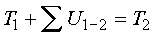The work-energy relation

Power of a force: Power in the ability of a force to do work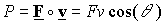F: The force applied on particle Q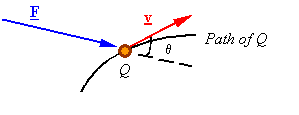v: The velocity of Q

Note: The power of a force is related to the velocity of the particle it is acting upon.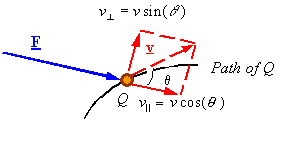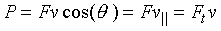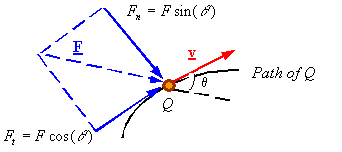Work of a force: The work U1-2 of a force on a particle over the interval of time from t1 to t2 is the integral of its power over this time interval.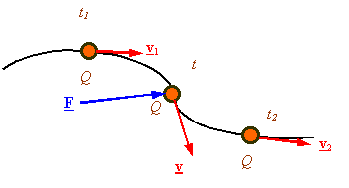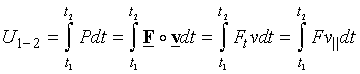Other methods to find the work of a force are: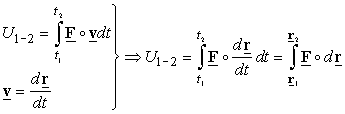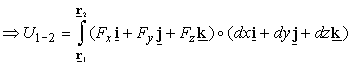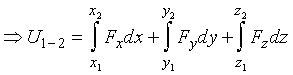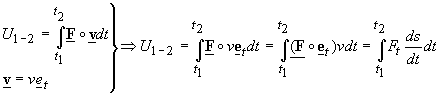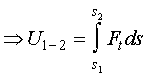Kinetic energy of a particle: A particle of mass m at each instant in time has a kinetic energy T given byThe work-energy relation: The relation between the work done on a particle by the forces which are applied on it and how its kinetic energy changes follows from Newton’s second law.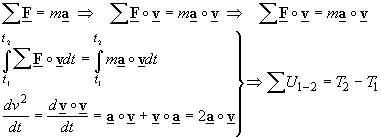Note: The work done on a particle by the resultant force applied on it over a given interval of time will be equal to the change in kinetic energy of the particle. In other words, the kinetic energy of a particle is changed by an amount equal to the work which is done on the particle by the resultant force.